# Round RoundUp RoundDown Floor Ceiling Int Even Odd

Rounding…, the cells in the selected range will be rounded in-place.

Rounding is especially useful when you want afuzzy matchbetween two tables. For example, if one tables column has a value 2.4, and the other tables column has a value 2.2, and you only care if the two values match in the integer part, then you can round up the 2 columns first, then do the match.Who else wants to do a 10-hour Excel analysis in 15 minutes?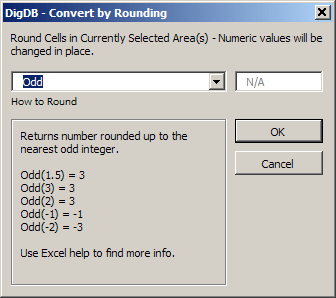RoundUp – rounds a number up, away from 0 (zero).ROUNDUP behaves like ROUND, except that it always rounds a number up.If number of digits is>

<0, then number is rounded up to the left of the decimal point. for example, rounding 31415.92654 by -2 digits equals 31,500.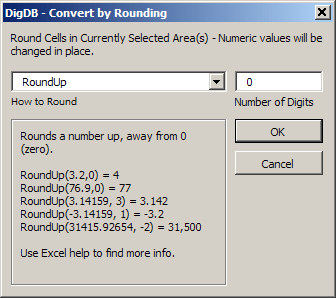EvenReturns number rounded up to the nearest even integer.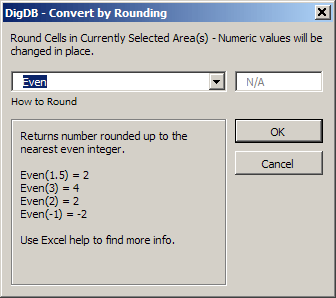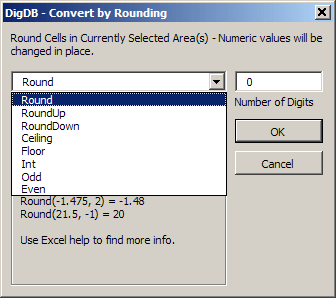Need more?Browse the complete list of DigDBs powerful features listed on thefront page.If you see errors or things not working, check out ourerror referencepage.

Round – rounds a number to a specified number of digits.If number of digits is>

free 15-day trialRounding values in a range or column in Excel -Round, RoundUp, RoundDown, Floor, Ceiling, Int, Even, OddExcel offers a variety of rounding formulas that allow a user to round a number to just about any approximation. Below is a list and rounding options. But the problem is that you cannot easily apply these formulas to an arbitrary range or table column. DigDB will allow you to do this:You can select a range, then invoke DigDB->

0 (zero), then number is rounded to the specified number of decimal places.If number of digits is = 0, then number is rounded to the nearest integer.If number of digits is

Selected Area->

IntRounds a number down to the nearest integer.

Practice file-rounding-demo.xls(16k)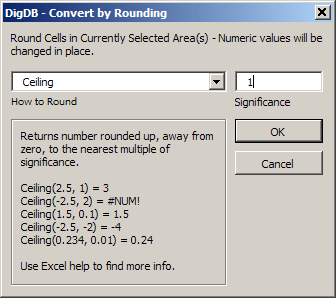Or, you can select a single cell in a table column, then invoke DigDB->

Microsoft Corporation in the United States and/or other countries.

In the rounding command window, use the dropdown box to select how to round. You have the following rounding options:

CeilingReturns number rounded up, away from zero, to the nearest multiple of significance. For example, if you want to avoid using pennies in your prices and your product is priced at \$4.42, CEILING 4.42 with significance of 0.05 will round prices up to the nearest nickel.

OddReturns number rounded up to the nearest odd integer.

Related keywords:Advanced excel tips, excel questions tools, Excel reporting applications,Excel add-insadd-in, Excel shareware, Excel plugin plug-ins plugins, Excel tricks question, Excel utility utilities,Excel add-onadd-ons, Excel tip tips, Power excel technique, Excel manipluation, Excel guru,Excel trick, Excel toolkit, Excel app power user

FloorRounds number down, toward zero, to the nearest multiple of significance.

Who else wants to do a 10-hour Excel analysis in 15 minutes?

0 (zero), then number is rounded up to the specified number of decimal places.If number of digits is = 0, then number is rounded up to the nearest integer.If number of digits is

<0, then number is rounded down to the left of the decimal point. for example, rounding 31415.92654 by -2 digits equals 31,400.0 (zero), then number is rounded down to the specified number of decimal places.If number of digits is = 0, then number is rounded down to the nearest integer.If number of digits is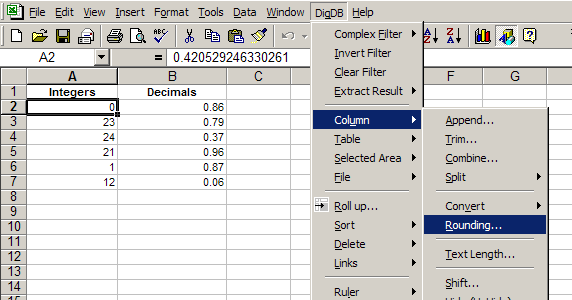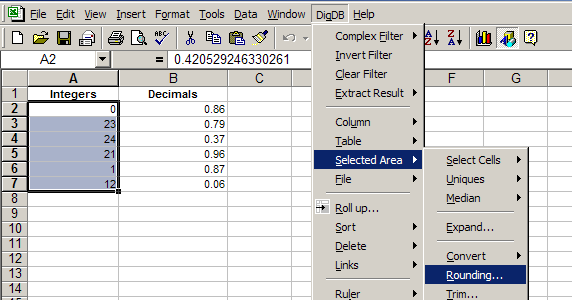Examples of Rounding results are shown when you select how to round.

Rounding…, the entire column will be rounded, and the rounded values will show in a new column inserted next to the original column.

RoundDown – rounds a number down, toward 0 (zero)ROUNDUP behaves like ROUND, except that it always rounds a number down.If number of digits is>

Column->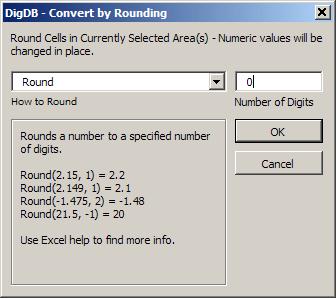<0, then number is rounded to the left of the decimal point. for example, rounding 21.5 by -1 digits equals to rounding the number to the nearest 10s, that is 20.